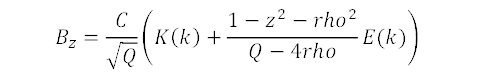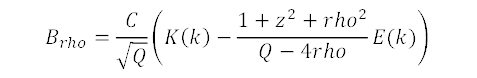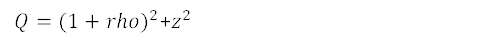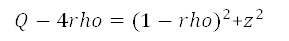# A calculation of the exact field near a loop of current

in an excel workbook using AGM and MAGM to calculate the needed elliptic integrals. As demonstrated by Semjon Adlaj , the Arithmetic Geometric Mean and a Modified Arithmetic Geometric Mean he discovered are by far the most efficient way to calculate Elliptic integral of the first and second kind.

## Vector representation

on the spreadsheet plot, the arrow length is (up to saturation) exactly the strength of the local magnetic field at the center of the arrow.

## Axes

The blue frame shows the range and position of the axes used in the plot that is in the workbook.Horizontally, the axis that is used is the cylindrical coordinate radius ρ from the axis of the unit radius current loop. The axis for ρ is from 0 to 1.9 in steps of 0.1.
Vertically, the axis that is used is the positive coordinate z along the axis of the unit radius current loop. The cylindrical coordinate z is ranging from 0 to 1.1 in steps of 0.1.
For negative z coordinates, the symmetry of the problem dictates the same field strength that the point at |z| and same ρ value. For those points, the radial component of the magnetic field Bρ becomes negative while Bz remains the same, the plane of the unit radius current loop thus acting as a kind of mirror. Because of this symmetry, we only need to show positive z coordinates.
The workbook contains worksheets for every elementary algebraic calculation. The symbol ρ is spelled rho due to constraints of Excel.
To accommodate the dimensions of the standard excel cell, increasing ρ values go in consecutive rows, while increasing z values go into increasing columns.

## Derivation

Refer to sources like http://www.kurtnalty.com/ClosedLoopFormulasForAandBwithCode.pdf

## Formulae

C being a constant proportional to the current in the unit radius loop, the axial and radial components of the magnetic field areandwhereandNote thatI've taken the radius to be unity but obviously all the results scale if you take a different radius.
Successive iterations of the AGM are calculated in the pairs of sheet {A,B},{C,D},{E,F},{G,H},...
Successive iterations of the MAGM are calculated in {L,M,N},{O,P,Q},{R,S,T},{U,V,W},...
after four AGM/MAGM iterations, results have converged to more (often, much more) than 7 decimal places.